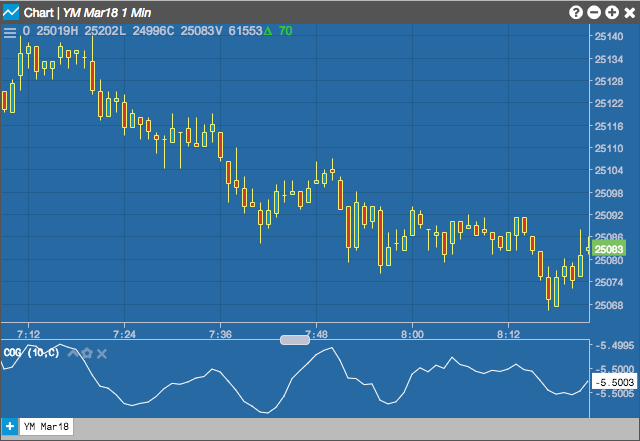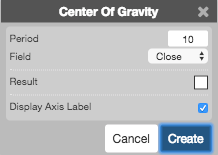Charts

# Center of Gravity (COG)

The Center of Gravity oscillator, developed by John Ehler, produces less lag indicating pivot points. The indicator was the result of studies of adaptive filters.## Configuration Options• Period: Number of days in the to use for calculations.
• Field: Price or combination of prices to use as the base for average calculations. Possible values include:
• Open
• High
• Low
• Close
• HL/2 $$\left ( \frac{High + Low}{2} \right )$$
• HLC/3 $$\left ( \frac{High + Low + Close}{3} \right )$$
• HLCC/4 $$\left ( \frac{High + Low + Close + Close}{4} \right )$$
• OHLC/4 $$\left ( \frac{Open + High + Low + Close}{4} \right )$$
$COG = \frac{-\sum P_{n}\times \left ( n+1 \right )}{\sum P_{n}}$